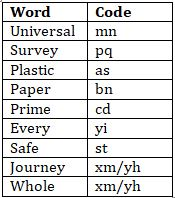Latest Banking jobs   »   Reasoning Ability Quiz For IBPS Clerk...

# Reasoning Ability Quiz For IBPS Clerk Prelims 2021- 9th August

Directions (1-5): Study the following information carefully and answer the given questions.
In a certain code language,
‘Every Survey universal plastic’ is coded as ‘yi pq mn as’
‘Paper safe Survey universal’ is coded as ‘mn bn st pq’
‘Paper prime universal plastic’ is coded as ‘cd as mn bn’
‘prime safe journey whole’ is coded as ‘cd st xm yh’

Q1. Which of the following is the code for ‘safe’?
(a) st
(b) bn
(c) pq
(d) mn
(e) None of these

Q2. Which of the following word is coded as ‘bn’?
(a) prime
(b) plastic
(c) safe
(d) paper
(e) None of these

Q3. What may be the code of ‘prime store’?
(a) st pq
(b) cd mn
(c) pq bn
(d) mn pq
(e) cd qw

Q4. Which of the following code is coded for ‘Survey’?
(a) mn
(b) pq
(c) bn
(d) cd
(e) None of these

Q5. What will be the code of ‘prime plastic’?
(a) st pq
(b) cd as
(c) cd pq
(d) bn as
(e) st bn

Q6. If in the number 67459138, 3 is subtracted to first five digit of the number and 1 is added to rest of the digits then how many digits are repeating in the number thus formed?
(a) Four
(b) One
(c) None
(d) three
(e) Two

Q7. Which of the following elements should come in a place of ‘?’
GJ11 MO16 RT21 ?
(a) WX26
(b) VX25
(c) WY26
(d) VY26
(e) None of these

Q8. How many pairs of letters are there in the word “ENDURANCE” each of which have as many letters between them in the word as they have between them in the English alphabetical series?
(a) Two
(b) One
(c) Three
(d) More than three
(e) None

Q9. Ravi is 22nd from the left end of a row and Hunny is 32th from the right end of row. If they interchanged their positions then ravi ranks become 21 from left end. How many persons sit between them?
(a) One
(b) Two
(c) None
(d) Three
(e) None of these

Q10. Among J, K, L, M and N, each of them is different weight. L’s weight is more than K. M’s weight is more than J and less than N. K is not the lightest person. M is not lighter than L. Who among them is the third heaviest?
(a) J
(b) L
(c) K
(d) M
(e) N

Directions (11-15): Following questions are based on the five three-digit numbers given below.

947 376 694 739 863

Q11. If all the digits in each of the numbers are arranged in increasing order within the number then, which of the following number will become the lowest in the new arrangement of numbers?
(a) 947
(b) 863
(c) 739
(d) 694
(e) 376

Q12. If all the numbers are arranged in ascending order from left to right then, which of the following will be the sum of all the three digits of the number which is 2nd from the right in the new arrangement?
(a) 18
(b) 19
(c) 15
(d) 16
(e) None of these

Q13. What will be the difference, when third digit of the 3rd lowest number is multiplied with the second digit of the highest number and third digit of the 2nd highest number is multiplied with the second digit of the lowest number?
(a) 21
(b) 20
(c) 15
(d)16
(e) None of these

Q14. If the positions of the second and the third digits of each of the numbers are interchanged then, how many even numbers will be formed?
(a) None
(b) One
(c) Two
(d) Three
(e) Four

Q15. If one is added to the second digit of each of the numbers and one is subtracted to the third digit of each number then, how many numbers thus formed will be divisible by three in new arrangement?
(a) None
(b) One
(c) Two
(d) Three
(e) Four

Solutions

Solutions (1-5):
Sol.S1. Ans. (a)
S2. Ans. (d)
S3. Ans. (e)
S4. Ans. (b)
S5. Ans. (b)

S6. Ans.(e)
Sol. Original number- 67459138
Obtained number- 34126249

S7. Ans.(c)

S8. Ans.(a)

S9. Ans(c)

S10. Ans(b)
N > M > L > K > J

S11.Ans(e)

S12.Ans(e)

S13.Ans(c)

S14.Ans(c)

S15.Ans(a)